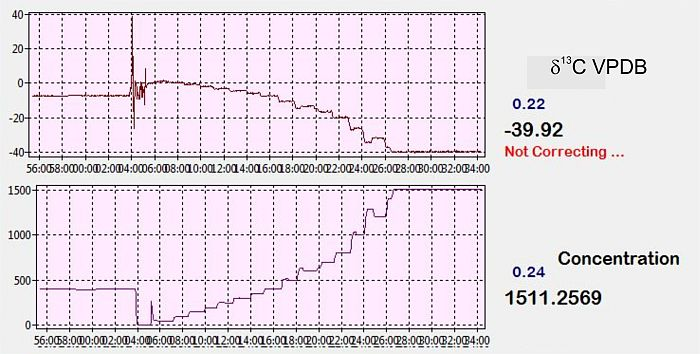# Data corrections 2 – Biting the bullet

First we need to think about how the basic differences from IRMS affect us.  One major difference is that we deal only in concentrations.  On an IRMS the ratio of the gas is everything.  If you want to calibrate to d18O = 85 permil VPDB then you need a gas with that ratio of 18O to 16O.  That’s not exactly true for a laser.  Let’s examine why.

If we run a CO2 with a d18O = +85 permil VPDB at 400 ppm for 626, then the concentration of 628 will be returned as about 434 ppm.   For 170 permil we would get  = 468.  Then we run at 1200 ppm for 626 and we get a value for 628 of 1302.   Increasing the CONCENTRATION can have a much greater effect than increasing the enrichment!  That means that we can scan across all reasonable values of  by changing concentration rather than by changing enrichment!  And the same applies to the other isotopologues.  We aren’t dealing with ratios anymore!  If the isotopologues are truly independent of each other then by scanning a concentration range, we are actually able to scan all the reasonable isotope enrichments!The concentration dependence is around 40 permil over our working range.  X on the graphs is Time while Y is concentration in the bottom graph and d13C in the upper.

What do we hope to pick up by these scans?  Well, if all the isotopologues concentrations started with zero offset and the reported concentrations all increased with a slope of exactly 1 as the analyte concentrations increased, then the answer is “Nothing useful”.  But that isn’t the case.  We know that there are strong concentration dependencies and weaker isotopic ones, implying that the reported concentrations do not have exactly identical slopes related to analyte concentration and/or that the initial offsets are slightly different.  Most of the problems probably relate to different slopes and possible curvature in the slopes: it would appear that all concentrations report zero at zero concentration.  But even the smallest offsets produce large differences in the deltas: take the error that you first thought of and multiply it by 1000 …Here a macro is used to run a concentration curve, with a correction function is loaded. The correction is failing slightly at high concentrations and the final return to 400 ppm does not produce the same delta as that concentration did earlier: there is a memory effect.  X is time, with Y as concentration in the lowest graph, then d13C above and d18O above that.

So we use our automated gas mixer to produce concentration ranges for CO2 of a known isotopic composition – perhaps from 100 ppm to 1100 ppm and we use these measurements to define the shape of the curves for all the reported isotopologues.  By using a single, known gas we can make the valid assumption that the isotopic ratio MUST be constant for all points.  And we can assume that each measured point IS correct.  Thus only the space between measured points remains somewhat uncertain.  But before continuing, there is one uncertainty even in these limitations: if you change the mixture of gases, then the concentration responses change.  Probably due to different band broadening.  This means that if you want to run experiments at 2% O2 and 21% O2 the you will definitely need to run separate concentration curves and calibrations.Concentration correction for d18O generated by program. The values are added to the raw laser analyses.

Another complication should be mentioned: if one concentration curve develops an offset from the other, perhaps due to slight non-linearity in the response, then the resulting delta curve can become just that: curved.  When plotted as a delta calculated from the ratios of the two isotopologues, the fit appears straight, although the R_squared might be .9999, when a straight line is subtracted the result is clearly curved.

The philosophy that Aerodyne proposes is to calculate the delta as (([minor_isotopologue]/[major_isotopologue])-1)*1000.  Thus if  = 403.5 and  = 400 then ((403.5/400)-1)*1000 = 8.75 permil VPDB.   Initially we used numbers to make our corrections.  Running two correction curves, with carbon dioxide gases with different compositions results in a grid of delta versus concentration.  We used this method for a while but it is not ideal over large ranges of delta or concentration.  The greatest difficulty is probably that if concentration is used as the X axis that the delta Y axis is not apparently linear.  If three different carbon dioxide gases are used to make three correction curves, with the three gases having compositions of 0, 20 and 40 permil, the distance on the Y axis between 0 and 20 is not the same as the distance from 20 to 40.  It is very difficult to know what function to use to interpolate between the curves or why it is necessary!  As a result of our experiments in this area, we decided that it was necessary to describe the laser responses better.